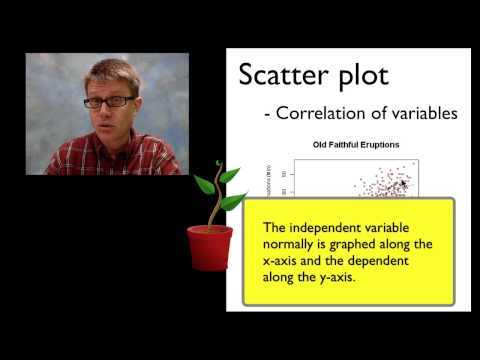### Graphing Bozeman# Graphing Bozeman

## 16 Video Interactions

1. 00:00:52

For our purposes the terms chart and graph mean the same thing.

• true

• false

2. 00:01:38

Approximately how much has CO2 levels changed since 1960?

• 70%

• 70 PPM

• 380 PPM
• Can't be determined

3. 00:03:06

Which graph do you use when looking at change over time?

• Bar graph

• histogram

• line graph

• pie

• scatter

4. 00:03:50

Which type of graph do you use when you are trying to see a correlation (relationship) between two sets of data?

• bar

• histogram

• line

• pie

• scatter

5. 00:04:12

Where is the independent variable located on a graph?

• x axis

• y axis

• within the graph

6. 00:04:24

Which variable is the one that displays the response to the variable that is being manipulated?

• dependent

• experimental

• independict

7. 00:04:27

The dependent variable is always placed on the Y axis of the graph

• true

• false

8. 00:04:39

Which type of graph is used to compare different groups?

• bar

• histogram

• line

• pie

• scatter

9. 00:05:28

Which type of graph is used to show the distribution of data?

• bar

• histogram

• line
• pie

• scatter

10. 00:05:37

Which type of graph is used to show parts to a whole?

• bar

• histogram

• line
• pie
• scatter

11. 00:06:41

The terms independent variable, manipulated variable, and responding variable all represent the same thing

• true

• fa;se

12. 00:07:45

The title on a graph should contain both the independent and dependent variable

• true

• false

13. 00:08:26

The distance between our data on the X and Y axis should be consistent.

• true

• false

14. 00:08:35

When appropriate the type of unit should be included on the X and Y axis

• true

• false

15. 00:08:47

All graphs must have the number 0 on it.

• true

• false

16. 00:10:15

The best fit line was incorrect because it extended beyond the data

• true

• false

Click here to preview, use, or make a copy of this interactive video lesson plan (bulb).